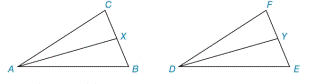Chapter 3.3, Problem 33E### Elementary Geometry for College St...

6th Edition
Daniel C. Alexander + 1 other
ISBN: 9781285195698

#### Solutions

Chapter
Section### Elementary Geometry for College St...

6th Edition
Daniel C. Alexander + 1 other
ISBN: 9781285195698
Textbook Problem
1 views

# Suppose that Δ A B C ≅ Δ D E F . Also, A X ¯ bisects ∠ C A B and D Y ¯ bisects ∠ F D E . Are these corresponding angle bisectors of congruent triangles congruent?Exercises 35, 36

To determine

To prove:

CAB is congruent to FDE.

Explanation

Approach:

(1) Corresponding parts of congruent triangles are congruent (CPCTC).

(2) If two angles and the included side of one triangle are congruent to two angles and the included side of a second triangle, then the triangles are congruent (ASA).

Calculation:

Consider the given figure.

Prove of the given statement is as follows.

 Proof Statement Reasons (1) ΔABC≅ΔDEF (1) Given (2) AC¯≅DF¯, AB¯≅

### Still sussing out bartleby?

Check out a sample textbook solution.

See a sample solution

#### The Solution to Your Study Problems

Bartleby provides explanations to thousands of textbook problems written by our experts, many with advanced degrees!

Get Started

#### [Type text] 2. If and ,are and are inverses? [Type text]

Mathematical Applications for the Management, Life, and Social Sciences

#### In Exercises 7-10, find the slope of the line shown in each figure. 8.

Applied Calculus for the Managerial, Life, and Social Sciences: A Brief Approach

#### True or False: .

Study Guide for Stewart's Single Variable Calculus: Early Transcendentals, 8th